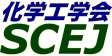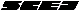## \$B9V1i%W%m%0%i%`!J%;%C%7%g%sJL!K(B

### \$B%7%s%]%8%&%`(B \$B!c

#### L100-L109, L113-L128, L200-L209, L213-L219, L300-L309, L313-L321

 \$B%*!<%,%J%\$%6!<(B \$BCf@n?B9%!J72GOBg!K!&IZ=E7=0l!JElKLBg!K!&ED4,9'7I!JEl9)Bg!K!&@>B<82!J;0=EBg!K!&>>1J7rB@O:!JEl

\$BG3NAEECS!"B@M[EECS!"Fs2A\$b=EMW\$G\$9!#K\%7%s%]%8%&%`\$G\$O!"G3NAEECS!"B@M[EECS!"Fs2AEy!K\$K4X\$9\$k9V1i\$r9-\$/Jg=8\$7!":`NA!"%W%m%;%9!"%7%9%F%`\$N8&5f\$K4p\$E\$/I}9-\$\$;kE@\$+\$i2=3X9)3X\$J\$i\$G\$O\$NF\$O@\$r9T\$\$\$^\$9!#\$^\$?!"K\%7%s%]%8%&%`\$O!"%(%M%k%.!]\$H\$7\$?I=>4\$r9T\$\$\$^\$9!#MW;]!&%W%l%<%s%F!<%7%g%s\$NL@2w\$5!"K\?M\$N4\$r9T\$\$\$^\$9!#3X@82q0w\$G2q0w?=@ACf\$NJ}\$OHw9MMs\$K\$=\$N;]5-:\\$/\$@\$5\$\$!#B?\$/\$NJ}!9\$N\$4;22C\$r\$*;}\$A\$7\$F\$*\$j\$^\$9!#(B

\$B:G=*99?7F|;~!'(B2012-08-20 15:02:17
\$B9V1i(B
\$B;~9o(B
\$B9V1i(B
\$BHV9f(B
\$B9V1iBjL\!?H/I=\$B%-!<%o!<%I(B\$BJ,N`(B
\$BHV9f(B
\$B\$BHV9f(B
L\$B2q>l(B \$BBh(B1\$BF|(B
(8:40\$B!A(B9:40)\$B!!(B(\$B:BD9(B \$B?\F#(B \$B2mIW(B)
8:40\$B!A(B 9:00L100PEFC\$BC1%;%k(Bin situ\$B29EYLLJ,I[B,Dj%G!<%?\$N:YJ,2=\$K\$h\$kEAG.FC@-I>2A\$HJ*
(\$B;0=EBg1!(B) \$B!{(B(\$B3X(B)\$B6aF#(B \$B90=S(B \$B!&(B (\$B@5(B)\$B@>B<(B \$B82(B \$B!&(B \$B;3Fb(B \$BBY2p(B \$B!&(B \$BHSHx(B \$BOB42(B \$B!&(B \$BW"ED(B \$B??;K(B
PEFC
In-plane temperature distribution
Impedance measurement
S-139
9:00\$B!A(B 9:20L101\$BM-5!(B-\$BL55!3&LL9bB.%W%m%H%sEAF38=>]\$rMxMQ\$7\$?8GBN9bJ,;R7AG3NAEECS\$N3+H/(B
(\$BEl9)Bg;q8;8&(B) \$B!{(B(\$B3X(B)\$B>.@n(B \$B7ILi(B \$B!&(B (\$B@5(B)\$BED4,(B \$B9'7I(B \$B!&(B (\$B@5(B)\$BBg66(B \$B=(Gl(B \$B!&(B (\$BElBg9)(B) \$B5m;3(B \$B9@(B \$B!&(B (\$B@5(B)\$B;32<(B \$B980l(B \$B!&(B (\$BEl9)Bg;q8;8&(B) (\$B@5(B)\$B;38}(B \$BLT1{(B
Fuel Cell
Proton conduction
an initio
S-153
9:20\$B!A(B 9:40L102\$B>.7?5!4oMQ(BPEFC\$B%9%?%C%/\$N7ZNL2=5Z\$SDc%3%9%H2=\$N8!F\$(B
(\$B90A0BgM}9)(B) \$B!{(B(\$B3X(B)\$BD%(B \$BD6(B \$B!&(B (\$B@D?98)9)Am8&(B) (\$B6&(B)\$B3k@>(B \$BM5(B \$B!&(B (\$B%S%C%H%F%C%/(B) \$BLZB<(B \$B1QM:(B \$B!&(B (\$B90A0Bg?7%(%M8&(B) (\$B@5(B)\$B41(B \$B9q@6(B \$B!&(B (\$B90A0BgM}9)(B) (\$B6&(B)\$B0KF#(B \$B> \$B!&(B (\$BElBg@88&(B) (\$B@5(B)\$BDi(B \$BFX;J(B \$B!&(B (\$B90A0Bg?7%(%M8&(B) (\$B@5(B)\$B0\$I[(B \$BN\$Ds(B

S-1110
(9:40\$B!A(B11:00)\$B!!(B(\$B:BD9(B \$B@>B<(B \$B82(B)
9:40\$B!A(B 10:00L103\$B8GBN9bJ,;R7AG3NAEECS1?E>;~\$N2a;@2=?eAG@8@.\$NI>2A(B
(\$B@EBg9)(B) \$B!{(B(\$B3X(B)\$B2,K\(B \$BM4 \$B!&(B (\$B3X(B)\$B>.Ln(B \$B8-;VO:(B \$B!&(B (\$B@5(B)\$B?\F#(B \$B2mIW(B
hydrogen peroxide
PEMFC
S-1354
10:00\$B!A(B 10:20L104\$BJ#6K@-Kl\$rMQ\$\$\$kG3NAEECS\$N1?E>FC@-(B
(\$B@EBg9)(B) \$B!{(B(\$B3X(B)\$B1J0f(B \$B=(OB(B \$B!&(B (\$B3X(B)\$B2<@n(B \$BN<2p(B \$B!&(B (\$B@EBg(B) (\$B@5(B)\$B?\F#(B \$B2mIW(B
Bipolar membrane
PEMFC
Plasma-Polymerization
S-1416
10:20\$B!A(B 10:40L105The Catalytic Properties of Nanoparticled Tantalum-based Catalysts by Electrodeposition under a Nonaqueous Plating Bath for Non-platinum Cathodes of PEFCs
(U. Tokyo) \$B!{(B(\$B3X(B)Seo J. \$B!&(B (KAUST) (\$B@5(B)Cha D. \$B!&(B (\$B@5(B)Takanabe K. \$B!&(B (U. Tokyo) (\$B@5(B)Kubota J. \$B!&(B (\$B@5(B)Domen K.
PEFC
Electrodeposition
Ta-based catalysts
S-1491
10:40\$B!A(B 11:00L106\$BJ.L84%AgK!\$KCf6u%]!<%i%9%+!<%\%sN3;R\$N9g@.\$HG3NAEECS?(G^\$X\$N1~MQ(B
(\$B9-Bg1!9)(B) \$B!{(B(\$B@5(B)\$B2.(B \$B?r(B \$B!&(B Ratna Balgis \$B!&(B (\$B@5(B)\$B1|;3(B \$B4n5WIW(B \$B!&(B (\$B%N%j%?%1%+%s%Q%K!<%j%_%F%I3+H/5;=QK\It(B) G. M. Anilkumar \$B!&(B \$B:89g(B \$B@!?M(B
Proton exchange membrane fuel cell
Electrocatalyst
spray drying
S-1638
(11:00\$B!A(B12:00)\$B!!(B(\$B;J2q(B \$BED4,(B \$B9'7I(B)
11:00\$B!A(B 12:00L107[\$B>7BT9V1i(B] \$BF|;:<+F0u\$HE8K>(B
(\$BF|;:<+F0
Polymer electrolyte fuel cell
S-1459
(13:00\$B!A(B14:20)\$B!!(B(\$B:BD9(B \$BJ!D9(B \$BGn(B)
13:00\$B!A(B 13:20L113\$BC:2=?eAG7OEE2r
(\$BEl5~;TBg(B) \$B!{(B(\$B3X(B)\$B6b;R(B \$BBYN4(B \$B!&(B \$BD9C+@n(B \$BK-(B \$B!&(B \$BFbCS(B \$BAo;V(B \$B!&(B (\$B@5(B)\$BIp(B \$BE/IW(B
Polymer Electrolyte Fuel Cell (PEFC)
Silica
Hydrocarbon membrane
S-1661
13:20\$B!A(B 13:40L114\$B0[\$J\$k%7%j%+8;\$K\$h\$jD4@=\$7\$?%7%j%+HoJ\$(BCNT\$BC4;}(BPt\$B%+%=!<%I?(G^\$N3h@-\$HBQ5W@-(B
(\$B6eBg1!9)(B) \$B!{(B(\$B3X(B)\$B1'ET5\(B \$BM5(B \$B!&(B \$B5\K\(B \$BBsL@(B \$B!&(B (\$B@5(B)\$BC]Cf(B \$BAT(B \$B!&(B (\$B@5(B)\$B>>:,(B \$B1Q \$B!&(B (\$B@5(B)\$B4_ED(B \$B>;9@(B
Pt catalyst
fuel cell
silica
S-1667
13:40\$B!A(B 14:00L115PEFC\$BMQ%+!<%\%s%J%N%A%e!<%VC4;}(BPd\$B%+%=!<%I?(G^\$NA+0\6bB0E:2C\$K\$h\$k?(G^3h@-8~>e(B
(\$B6eBg1!9)(B) \$B!{(B(\$B3X(B)\$BDMK\(B \$BCR@2(B \$B!&(B (\$B@5(B)\$BC]Cf(B \$BAT(B \$B!&(B (\$B@5(B)\$B>>:,(B \$B1Q \$B!&(B (\$B@5(B)\$B4_ED(B \$B>;9@(B
PEFC
Pd alloy catalyst
oxygen reduction reaction
S-1685
14:00\$B!A(B 14:20L116\$B%W%m%H%sEAF3@-%j%s;@%,%i%9\$N9g@.\$HEE5\$EAF3FC@-(B
(\$BElBg1!?7NN0h(B) \$B!{(B(\$B3X(B)\$B@nB<(B \$BN \$B!&(B (\$B@5(B)\$BBgM'(B \$B=g0lO:(B \$B!&(B (\$B@5(B)\$BBgEg(B \$B5A?M(B
fuel cell
proton conducting glass material
sol-gel method
S-1765
(14:20\$B!A(B15:40)\$B!!(B(\$B:BD9(B \$BC]Cf(B \$BAT(B)
14:20\$B!A(B 14:40L117Nafion\$B%-%c%C%T%s%0BN\$rMQ\$\$\$?EE6K?(G^AX\$N?(G^MxMQN(8~>e\$K8~\$1\$?8!F\$(B
(\$BEl9)Bg;q8;8&(B) \$B!{(B(\$B3X(B)\$BCfEg(B \$BC#:H(B \$B!&(B (\$B@5(B)\$BED4,(B \$B9'7I(B \$B!&(B (\$B@5(B)\$BBg66(B \$B=(Gl(B \$B!&(B (\$B@5(B)\$B;38}(B \$BLT1{(B
PEFC
Catalyst layer
Nafion Capping
S-1820
14:40\$B!A(B 15:00L118\$B%"%s%b%K%"=hM}\$7\$?%7%k%/3h@-C:\$r%+%=!<%I\$KMQ\$\$\$?(BPEFC\$B\$NH/EEFC@-(B
(\$B?.=#BgA!0](B) \$B!{(B(\$B@5(B)\$BJ!D9(B \$BGn(B \$B!&(B \$BBg;3(B \$B63J?(B \$B!&(B (\$B@5(B)\$B9b66(B \$B?-1Q(B \$B!&(B (\$B@5(B)\$B9bDM(B \$BF)(B \$B!&(B (\$BBgF|K\0u:~(B) (\$B@5(B)\$B4_K\(B \$BHfO\$;V(B
PEFC
non-precious metal catalysts
carbon
S-1956
15:00\$B!A(B 15:20L119\$B9g6bI=LL\$KD>@\9g@.\$7\$?%+!<%\%s%J%N%A%e!<%V\$N%0%k%3!<%9G3NAEECSEE6K\$X\$N1~MQ(B
(\$B5~Bg9)(B) \$B!{(B(\$B3X(B)\$B;y6L(B \$B9dIp(B \$B!&(B (\$B@5(B)\$B:4Ln(B \$B5*>4(B \$B!&(B (\$B@5(B)\$BEDLg(B \$BH%(B
fuel cell
carbon nanotube
chemical vapor deposition
S-17
15:20\$B!A(B 15:40L120A Facile Thermal Method for Producing Novel 3-D Graphite Oxide-Nanoribbon Supported Metal Electrocatalysts for DMFC
(\$BEl9)Bg;q8;8&(B) \$B!{(B(\$B3X(B)\$B]j(B \$B3\$NS(B \$B!&(B (\$B@5(B)Kakade B. A. \$B!&(B (\$B@5(B)\$BED4,(B \$B9'7I(B \$B!&(B (\$B@5(B)\$BBg66(B \$B=(Gl(B \$B!&(B (\$B@5(B)\$B;38}(B \$BLT1{(B
DMFC
methanol oxidation reaction
graphite oxide, nanoribbon
S-1298
(15:40\$B!A(B17:00)\$B!!(B(\$B:BD9(B \$B;38}(B \$BLT1{(B)
15:40\$B!A(B 16:00L121\$B9bG;EY%a%?%N!<%k\$r;HMQ\$9\$kD>@\%a%?%N!<%k7?G3NAEECS\$NH/EEFC@-(B
(\$B72Bg1!9)(B) \$B!{(B(\$B3X(B)\$B]/0f(B \$B=!(B \$B!&(B (\$B@5(B)\$BDT8}(B \$BBsLi(B \$B!&(B (\$B@5(B)\$BCf@n(B \$B?B9%(B
direct methanol fuel cell (DMFC)
boiling point
air humidity
S-1302
16:00\$B!A(B 16:20L122\$B%O%\$%Q!<%V%i%s%A%]%j%^!<\$rMQ\$\$\$?D>@\%.;@7?G3NAEECS\$NEE6K:n@=J}K!\$N8!F\$(B
(\$B72Bg1!9)(B) \$B!{(B(\$B@5(B)\$BDT8}(B \$BBsLi(B \$B!&(B (\$B3X(B)\$B4d>e(B \$BM@6k(B \$B!&(B (\$B3X(B)\$B>.V:(B \$BL((B \$B!&(B (\$B@5(B)\$BCf@n(B \$B?B9%(B \$B!&(B (\$BF|;:2=3X(B) \$B>.Eg(B \$B7=2p(B
DFAFC
Hyper branched-polymer
Stablizer
S-1458
16:20\$B!A(B 16:40L123\$BD>@\%.;@7?G3NAEECS\$NFC0[\$J%+%=!<%I2aEE05\$NA}2C(B
(\$B72Bg1!9)(B) \$B!{(B(\$B3X(B)\$B4d>e(B \$BM@6k(B \$B!&(B (\$B@5(B)\$BDT8}(B \$BBsLi(B \$B!&(B (\$B@5(B)\$BCf@n(B \$B?B9%(B
DFAFC
Cathode overvoltage
Low concentration
S-1561
16:40\$B!A(B 17:00L124TiO2\$B4^M-(BCNF\$BC4;}(BPtRu\$B?(G^\$N%"%k%3!<%k;@2=FC@-(B
(\$B72Bg1!9)(B) \$B!{(B(\$B3X(B)\$B0KF#(B \$BM:Bg(B \$B!&(B (\$B@5(B)Abdelkareem Mohammad Ali \$B!&(B (\$B@5(B)\$BDT8}(B \$BBsLi(B \$B!&(B (\$B@5(B)\$BCf@n(B \$B?B9%(B
DAFC
catalyst
TiO2
S-1679
(17:00\$B!A(B18:20)\$B!!(B(\$B:BD9(B \$BCf@n(B \$B?B9%(B)
17:00\$B!A(B 17:20L125\$BM}A[E*(BOH-\$BEAF3%A%c%M%k\$NC5:w\$K8~\$1\$?J,;R%G%6%\$%s7?5!G=@-:`NA\$N3+H/(B
(\$BEl9)Bg;q8;8&(B) \$B!{(B(\$B3X(B)\$BD9C+@n(B \$B00(B \$B!&(B \$B>1;R(B \$BNI98(B \$B!&(B \$BJ!Eg(B \$B9'E5(B \$B!&(B (\$B@5(B)\$BBg66(B \$B=(Gl(B \$B!&(B (\$B@5(B)\$BED4,(B \$B9'7I(B \$B!&(B (\$B@5(B)\$B;38}(B \$BLT1{(B
SAFC
micro phase separation
anion exchange membrane
S-1823
17:20\$B!A(B 17:40L126\$BA48GBN7?%"%k%+%jG3NAEECSMQEE2r
(\$BEl9)Bg;q8;8&(B) \$B!{(B(\$B3X(B)\$BCz(B \$B9aH~(B \$B!&(B (\$B@5(B)\$BBg66(B \$B=(Gl(B \$B!&(B (\$B@5(B)\$BED4,(B \$B9'7I(B \$B!&(B (\$B@5(B)\$B;38}(B \$BLT1{(B
fuel cell
Layered double hydroxide
anion conductor
S-1836
17:40\$B!A(B 18:00L127\$B:Y9&%U%#%j%s%07?%"%K%*%s8r49Kl\$NFC@-\$HH/EE;~?e0\F0\$N2r@O(B
(\$BEl9)Bg;q8;8&(B) \$B!{(B(\$B3X(B)\$BD%(B \$B^>(B \$B!&(B (\$B@5(B)\$BBg66(B \$B=(Gl(B \$B!&(B (\$B@5(B)\$BED4,(B \$B9'7I(B \$B!&(B (\$B@5(B)\$B;38}(B \$BLT1{(B
Anion exchange membrane
Water movement
Pore filling membrane
S-1847
18:00\$B!A(B 18:20L128\$BCf29:nF0%W%m%H%sEAF37?G3NAEECS\$K\$*\$1\$k3F
(\$BElBg1!?7NN0h(B) \$B!{(B(\$B3X(B)\$BEhED(B \$B8^I4N\$(B \$B!&(B (\$B@5(B)\$BBgEg(B \$B5A?M(B \$B!&(B (\$B@5(B)\$BBgM'(B \$B=g0lO:(B
intermediate temperature fuel cell
proton conductor
anode reaction
S-1547
L\$B2q>l(B \$BBh(B2\$BF|(B
(8:40\$B!A(B9:40)\$B!!(B(\$B:BD9(B \$B5FCO(B \$BN4;J(B)
8:40\$B!A(B 9:00L200\$B%j%A%c!<%8%c%V%k!&%=%j%C%I%+!<%\%sG3NAEECS\$K\$*\$1\$kH/EEFC@-\$NG3NA0MB8\$H%a%+%K%:%`(B
(\$BEl9)Bg2=3X(B) \$B!{(B(\$B3X(B)\$BDD(B \$B1P(B \$B!&(B (\$B3X(B)\$BLp?a(B \$BL@K!(B \$B!&(B (\$B3X(B)\$BEDCf(B \$BM5 \$B!&(B (\$B@5(B)\$B0K86(B \$B3X(B
fuel cells
SOFC
direct carbon
S-1986
9:00\$B!A(B 9:20L201\$B%j%A%c(B-\$B%8%c%V%k!&(BDCFC\$B\$K\$*\$1\$kG3NA6KH?1~%5%\$%/%k\$X\$N1F6AMW0x\$N8!F\$(B
(\$BEl9)Bg2=3X(B) \$B!{(B(\$B3X(B)\$BEDCf(B \$BM5 \$B!&(B (\$B3X(B)\$BLp?a(B \$BL@K!(B \$B!&(B (\$B3X(B)\$BM{(B \$B?71'(B \$B!&(B (\$B3X(B)\$BDD(B \$B1P(B \$B!&(B (\$B@5(B)\$B0K86(B \$B3X(B
solid oxide
fuel cell
direct cabon
S-1988
9:20\$B!A(B 9:40L202\$B8GBN;@2=J*7AG3NAEECS\$K\$*\$1\$kC:2=?eAGMxMQ\$K8~\$1\$?7W;;2=3X2r@O(B
(\$B6eBg0p@9%;(B/\$B6eBg(BI2CNER/JST-CREST) \$B!{(B(\$B@5(B)\$B8E;3(B \$BDL5W(B \$B!&(B (\$B6eBg1!9)(B) \$BED86(B \$B=S2p(B \$B!&(B (\$B6eBg1!9)(B/\$B3X?6(B) \$BCfHx(B \$BOB1Q(B \$B!&(B (\$B6eBg0p@9%;(B/JST-CREST) Saha Leton \$B!&(B (\$B@5(B)\$B@P85(B \$B9'2B(B
Solid Oxide Fuel Cell
Hydrocarbon
Anode
S-1166
(9:40\$B!A(B11:00)\$B!!(B(\$B:BD9(B \$BBgM'(B \$B=g0lO:(B)
9:40\$B!A(B 10:00L203\$B%+!<%\%sE:2CJ.L8G.J,2rK!\$K\$h\$k(BSOFC\$B:`NA%J%NN3;R\$N9g@.(B
(\$B:eI\Bg1!9)(B) \$B!{(B(\$B3X(B)\$B%"%i%9%H(B \$B%"%_%k%l%6(B \$B!&(B (\$B@5(B)\$BLZ2<(B \$BBnLi(B \$B!&(B (\$B@5(B)\$BB-N)(B \$B85L@(B
spray pyrolysis
SOFC
electrolyte
S-1204
10:00\$B!A(B 10:20L204\$BFs
(\$B:eI\Bg9)(B) \$B!{(B(\$B3X(B)\$BH+;3(B \$BNN(B \$B!&(B (\$B@5(B)\$BG_:j(B \$BM'Lp(B \$B!&(B (\$B3X(B)\$B8~0f(B \$B7r?M(B \$B!&(B (\$B@5(B)\$BDE5W0f(B \$BLP \$B!&(B (\$B@5(B)\$BB-N)(B \$B85L@(B \$B!&(B (\$B:eBg;:8&(B) (\$B@5(B)\$B0f \$B!&(B (\$B@5(B)\$BB?:,(B \$B@5OB(B \$B!&(B (\$B@5(B)\$BCfEh(B \$B1QM:(B
SOFC
SIMS
Diffusion
S-1249
10:20\$B!A(B 10:40L205\$BAX>u%Z%m%V%9%+%\$%H;@2=J*(BPr1-xM1+xInO4(M=Ba, Sr)\$B\$rEE2r
(\$BEl9)Bg2=3X(B) \$B!{(B(\$B3X(B)\$BM{(B \$B?71'(B \$B!&(B (\$B3X(B)\$BEgED(B \$B42G7(B \$B!&(B (\$B@5(B)\$B0K86(B \$B3X(B
Solid oxide fuel cell
electrolyte
ionic conductor
S-1309
10:40\$B!A(B 11:00L206\$B:.9gEAF3BN\$rMQ\$\$\$?(BSOFC\$BG3NA6K\$N8&5f(B
(\$BElBg1!9)(B) \$B!{(B(\$B3X(B)\$BFn(B \$BC\$;V(B \$B!&(B (\$B@5(B)\$B5FCO(B \$BN4;J(B \$B!&(B (\$B@5(B)\$B9b3@(B \$BFX(B \$B!&(B (\$B@5(B)\$B?{86(B \$B9'(B \$B!&(B Oyama S. T.
SOFC
anode
mixed ionic and electronic conductor
S-1355
(11:00\$B!A(B12:00)\$B!!(B(\$B;J2q(B \$BIZ=E(B \$B7=0l(B)
11:00\$B!A(B 12:00L207[\$B>7BT9V1i(B]\$B8GBN;@2=J*7AG3NAEECS(B(SOFC)\$B\$N3+H/F08~\$HNt2=BP:v(B
(\$B5~Bg(B) \$B!{9>8}(B \$B9@0l(B
SOFC
S-1111
(13:00\$B!A(B14:40)\$B!!(B(\$B:BD9(B \$B0K86(B \$B3X(B)
13:00\$B!A(B 13:20L213Suitable operational strategy to utilize wasted heat from micro-CHP systems
(Kyushu U.) \$B!{(B(\$B3X(B)Taufiq B. N. \$B!&(B (\$B@5(B)Ishimoto T. \$B!&(B (\$B@5(B)Koyama M.
Micro-CHP
Heat pump
CO2 emission
S-1477
13:20\$B!A(B 13:40L214Numerical Investigations of a Novel Type of Micro-tubular Solid Oxide Fuel Cell
(Inst. Industrial Sci., The U. Tokyo) \$B!{(B(\$B3X(B)Panthi Dhruba \$B!&(B (\$B@5(B)Tsutsumi Atsushi
Solid Oxide Fuel Cell
Micro-tubular Design
Computational Fluid Dynamics
S-1689
13:40\$B!A(B 14:00L215SOFC\$B6u5\$6K\$K\$*\$1\$k@=B\$%W%m%;%9M3MhHyNL@.J,\$NEE6KFC@-\$KBP\$9\$k1F6AI>2A(B
(\$BElBg1!?7NN0h(B) \$B!{(B(\$B3X(B)\$BBg@P(B \$B=_Lp(B \$B!&(B (\$B@5(B)\$BBgEg(B \$B5A?M(B \$B!&(B (\$B@5(B)\$BBgM'(B \$B=g0lO:(B
solid oxide fuel cell
minor components
electrode property
S-1764
14:00\$B!A(B 14:20L216SOFC\$BEE2r=@-\$H=PNO\$NAj4X(B
(\$B:eI\Bg9)(B) \$B!{(B(\$B3X(B)\$B8~0f(B \$B7r?M(B \$B!&(B (\$B3X(B)\$BH+;3(B \$BNN(B \$B!&(B (\$B@5(B)\$BDE5W0f(B \$BLP \$B!&(B (\$BETN);:5;9b@l(B) (\$B@5(B)\$B5HED(B \$B7r0l(B \$B!&(B (\$B:eI\Bg9)(B) \$B@P66(B \$B9-5-(B \$B!&(B (\$B@5(B)\$BB-N)(B \$B85L@(B \$B!&(B (\$B;:5;8&(B) \$Bd*(B \$BK'<#(B \$B!&(B \$B:4F#(B \$BOBO:(B \$B!&(B \$BF|2<(B \$BCi6=(B
SOFC
PLD
Electrolyte
S-1771
14:20\$B!A(B 14:40L217\$B:n@=K!\$N0[\$J\$k(BSOFC\$BMQ%Z%m%V%9%+%\$%H7?;@2=J*%"%N!<%I\$NH/EEFC@-(B
(\$B?.=#BgA!0](B) \$B!{(B(\$B3X(B)\$BEOn5(B \$B85(B \$B!&(B \$B>>;3(B \$BIp;V(B \$B!&(B (\$B@5(B)\$B9b66(B \$B?-1Q(B \$B!&(B (\$B@5(B)\$B9bDM(B \$BF)(B \$B!&(B (\$B@5(B)\$BJ!D9(B \$BGn(B
solid oxide fuel cell
anode
perovskite
S-1913
(14:40\$B!A(B15:20)\$B!!(B(\$B:BD9(B \$BED4,(B \$B9'7I(B)
14:40\$B!A(B 15:00L218\$B:F@8C:AGA!0]\$N%j%5%\$%/%k5;=Q(B
(\$B4tIlBg9)(B) \$B!{(B(\$B3X(B)\$B@>B<(B \$B8-Bg(B \$B!&(B (\$B@5(B)\$B \$B!&(B (\$B@5(B)\$B7(It(B \$BOB90(B
CFRP
calcination
pyrolysis
S-1656
15:00\$B!A(B 15:20L219\$B%j%5%\$%/%kC:AGA!0]\$N6/EYI>2AJ}K!(B
(\$B4tIlBg9)(B) \$B!{(B(\$B3X(B)\$B2CF#(B \$B9,@8(B \$B!&(B (\$B@5(B)\$B \$B!&(B (\$B@5(B)\$B?@86(B \$B?.;V(B \$B!&(B (\$B@5(B)\$B7(It(B \$BOB90(B
Carbon fiber
Tensile strength
Tensile modulus
S-1666
L\$B2q>l(B \$BBh(B3\$BF|(B
(8:40\$B!A(B10:00)\$B!!(B(\$B:BD9(B \$B2O@%(B \$B85L@(B)
8:40\$B!A(B 9:00L300\$B%a%?%N!<%k%*!<%H%5!<%^%k2~CHq\$K4X\$9\$k8&5f(B
(\$B7DXfBg(B) \$B!{(B(\$B3X(B)\$BBgC+(B \$B=a(B \$B!&(B \$BOBED(B \$B>;>M(B \$B!&(B M. F. Hasan \$B!&(B (\$B@5(B)\$B2#?9(B \$B9d(B \$B!&(B (\$B@5(B)\$B?"ED(B \$BMx5W(B
Reforming
Hydrogen
Auto-Thermal
S-1224
9:00\$B!A(B 9:20L301\$B%*%U%,%9:F=[4D\$rH<\$&%*!<%H%5!<%^%k2~
(\$B7DXfBgM}9)(B) \$B!{(B(\$B3X(B)\$B;~G\$(B \$B:L(B \$B!&(B (\$B3X(B)\$B@uLn(B \$B;J(B \$B!&(B (\$B3X(B)Hasan M. F. \$B!&(B (\$B@5(B)\$B2#?9(B \$B9d(B \$B!&(B (\$B@5(B)\$B?"ED(B \$BMx5W(B
reformer
autothermal
off-gas recirculation
S-1237
9:20\$B!A(B 9:40L302\$BDLEE2CG.%"%k%^%\$%H?(G^\$rMQ\$\$\$?%a%?%s?e>x5\$2~
(\$BElG@9)Bg(B) \$B!{(B(\$B3X(B)\$B1|Ln(B \$B@i1{(B \$B!&(B (\$B3X(B)\$BD%(B \$B7D51(B \$B!&(B (\$B@5(B)\$B3T(B \$B%f(B \$B!&(B (\$B@5(B)\$B55;3(B \$B=(M:(B \$B!&(B (\$B@5(B)\$B:y0f(B \$B@?(B
Steam reforming
Anodic alumina support
Nickel catalyst
S-1389
9:40\$B!A(B 10:00L303\$B%j%s;@7AG3NAEECS%7%9%F%`\$N;@2=H?1~4o\$H\$7\$F\$N1~MQ(B
(\$BL>Bg1!9)(B) \$B!{(B(\$B3X(B)\$B2->e(B \$B?? \$B!&(B (\$B@5(B)\$B;3ED(B \$BGn;K(B \$B!&(B (\$B@5(B)\$BED@n(B \$BCRI'(B \$B!&(B (\$BL>;T9)8&(B) (\$B@5(B)\$B5\ED(B \$B9/;K(B
Fuel cell reactor
PAFC
Selecteve oxidation
S-1840
(10:00\$B!A(B11:00)\$B!!(B(\$B:BD9(B \$B8E;3(B \$BDL5W(B)
10:00\$B!A(B 10:20L304\$BDX303L\$r86NA\$H\$9\$k%a%=9&C:AG\$ND4@=\$H%-%c%Q%7%?FC@-(B
(\$B:eI\Bg1!(B) \$B!{(B(\$B3X(B)\$BBgC+(B \$B=S2p(B \$B!&(B (\$B@5(B)\$BIpF#(B \$BL@FA(B
capacitor
mesopore
carbon
S-1675
10:20\$B!A(B 10:40L305\$B%0%i%U%'%s\$NMO1U9g@.!"%J%N9=B\$@)8f\$HBgMFNL%-%c%Q%7%?\$X\$N1~MQ(B
(\$BElKLBgB?858&(B) \$B!{;0C+(B \$BM!(B \$B!&(B \$B%^%i%Q%s(B \$B%5%F%#%C%7%e(B \$B!&(B \$B1':,K\(B \$BFF(B \$B!&(B \$Bcx5o(B \$B9bL@(B \$B!&(B (\$B@5(B)\$BK\4V(B \$B3J(B
Graphene
Capacitor
Electrode
S-1466
10:40\$B!A(B 11:00L306\$B%^%\$%/%m%A%c%M%k\$r7A@.\$7\$?9=B\$2=EE6K\$K\$h\$kEE5\$Fs=EAX%-%c%Q%7%?\$NB.EY@-G=8~>e(B
(\$B5~Bg9)(B) \$B!{(B(\$B3X(B)\$BBgAR(B \$B:M>:(B \$B!&(B (\$B3X(B)\$B \$B!&(B (\$B@5(B)\$B0f>e(B \$B85(B \$B!&(B (\$B@5(B)\$B2O@%(B \$B85L@(B
Electrochemical double-layer capacitor
Structured electrode
High discharge rate
S-183
(11:00\$B!A(B12:00)\$B!!(B(\$B:BD9(B \$B0f>e(B \$B85(B)
11:00\$B!A(B 11:20L307\$BHyJ4%;%k%m!<%9!A%]%j%"%/%j%k%K%H%j%kJ#9gA!0]\$NC:AG:`NA2=\$H%j%A%&%`%\$%*%s%-%c%Q%7%?\$X\$NE,MQ(B
(\$B:eI\Bg1!9)(B) \$B!{(B(\$B3X(B)\$B?eLn(B \$B5.A1(B \$B!&(B (\$B@5(B)\$BIpF#(B \$BL@FA(B \$B!&(B (\$B2,;3Bg(B) \$B2-86(B \$B9*(B \$B!&(B \$BLZ2<(B \$B72Kc(B \$B!&(B (\$BF|K\%(%/%9%i%s(B) \$BBgOB(B \$B2B5V(B
lithium ion capacitor
polyacrylonitrile
cellulose
S-1284
11:20\$B!A(B 11:40L308\$B%j%A%&%`%\$%*%sEECS\$K\$*\$1\$k3hJ*
(\$B6bBtBg1!<+(B) \$B!{(B(\$B3X(B)\$BC#@n(B \$B1Q<#(B \$B!&(B \$BCSED(B \$BCR=((B \$B!&(B (\$B6bBtBgM}9)(B) (\$B@5(B)\$BEDB<(B \$BOB90(B
Lithium-ion battery
Intercalation electrode
Diffusion process
S-1898
11:40\$B!A(B 12:00L309\$BL5MOG^H?1~\$rMQ\$\$\$??7\$7\$\$%]%j%^!<9g@.\$H%j%A%&%`Fs
(\$BJF;R9b@l(B) \$B!{(B(\$B3X(B)\$B%h%O%M%9(B \$B%/%j%9%F%#(B \$B!&(B (\$B3X(B)\$B@.ED(B \$B5.8w(B \$B!&(B (\$BJF;R9b@l@l962J(B) \$Bzp66(B \$BBg \$B!&(B (\$BJF;R9b@l(B) \$B>>0f(B \$B0jLi(B \$B!&(B \$B@V0f(B \$BBg5\$(B \$B!&(B \$BC]Fb(B \$BM4B@(B \$B!&(B \$BJ?2,(B \$BBsMN(B \$B!&(B (\$B@5(B)\$BC+F#(B \$B>05.(B \$B!&(B (\$BL>Bg1!M}(B) \$B5H@n(B \$B9@;K(B
non solvent reaction
disulfide
lithium rechargable battery
S-1213
(13:00\$B!A(B13:40)\$B!!(B(\$B;J2q(B \$BCf@n(B \$B?B9%(B)
13:00\$B!A(B 13:40L313[\$BE8K>9V1i(B]\$B%j%A%&%`%\$%*%sFs
(\$BEl9)Bg(B) \$B!{(B(\$B@5(B)\$BC+8}(B \$B@t(B
LIB
Cathode materials
Fine particles
S-1102
(13:40\$B!A(B14:20)\$B!!(B(\$B:BD9(B \$B8E;3(B \$BDL5W(B)
13:40\$B!A(B 14:00L315\$B%\$%*%s1UBN4^M-5?;w8GBNEE2r2A(B
(\$BElKLBgB?858&(B) \$B!{(B(\$B@5(B)\$B1':,K\(B \$BFF(B \$B!&(B \$B>>Hx(B \$BN48<(B \$B!&(B \$B>.@n(B \$B=(G7(B \$B!&(B \$B4gIt(B \$B>M9T(B \$B!&(B (\$B@5(B)\$BK\4V(B \$B3J(B
All-solid-state battery
Ionic Liquid
Mass transport
S-1543
14:00\$B!A(B 14:20L316\$B%\$%*%s1UBN4^M-5?;w8GBNEE2r
(\$BElKLBgB?858&(B) \$B!{4gIt(B \$B>M9T(B \$B!&(B \$B>.@n(B \$B=(G7(B \$B!&(B (\$B@5(B)\$B1':,K\(B \$BFF(B \$B!&(B (\$B@5(B)\$BK\4V(B \$B3J(B
All-solid-state battery
Ionic Liquid
High capacity cathode
S-1545
(14:20\$B!A(B16:00)\$B!!(B(\$B:BD9(B \$B1':,K\(B \$BFF(B)
14:20\$B!A(B 14:40L317(\$B9V1iCf;_(B)

100526
14:40\$B!A(B 15:00L318\$B%_%/%m!&%J%N%/%i%9%?!<9=B\$2=\$K\$h\$k6bB0%J%NN3;RKl\$N:n@.\$HB?@\9gGvKlB@M[EECS\$X\$N1~MQ(B
(\$BEl9)Bg2=3X(B) \$B!{(B(\$B3X(B)\$BFn(B \$B41M4(B \$B!&(B (\$B3X(B)\$B5H2,(B \$BfFJ?(B \$B!&(B (\$B@5(B)\$B0K86(B \$B3X(B
Solar cells
Metal nanoparticles
Surface plasmon
S-1755
15:00\$B!A(B 15:20L319Sb2S3\$BA}46H>F3BNB@M[EECS\$K\$*\$1\$k8wA}46:`\$N(Bpn\$B@\9g3&LL\$X\$NC4;}>uBV\$HH/EEFC@-\$N4X78(B
(\$BEl9)Bg2=3X(B) \$B!{(B(\$B3X(B)\$B5H2,(B \$BfFJ?(B \$B!&(B \$B;0Eg(B \$B?rDw(B \$B!&(B (\$BIt(B)\$B0K86(B \$B3X(B
solar cell
Sb2S3
heterojunction
S-1942
15:20\$B!A(B 15:40L320Interaction of Metal Nanoparticles and TiO2 during the Introduction into the Photoabsorbing Layer in Plasmonic Dye-Sensitized Solar Cells
(\$BEl9)Bg2=3X(B) \$B!{(B(\$B@5(B)Loew Noya \$B!&(B (\$B@5(B)\$B0K86(B \$B3X(B
dye-sensitized solar cell
metal nanoparticle
localized surface plasmon
S-1944
15:40\$B!A(B 16:00L321\$BD>8rN.7?29EY:9H/EEAuCV\$N@-G=I>2A(B
(\$BIY;39bEy@lLg3X9;(B) \$B!{(B(\$B@5(B)\$B5H@n(B \$BJ87C(B \$B!&(B (\$B@5(B)\$BCz;R(B \$BE/<#(B \$B!&(B \$BEg(B \$B@/;J(B \$B!&(B \$B>._7(B \$BL/;R(B \$B!&(B \$B>e:d(B \$B@](B
Thermoelectric Power Generation
Design Optimization
Heat Transfer
S-1218

\$B9V1i%W%m%0%i%`(B
\$B2=3X9)3X2q(B \$BBh(B44\$B2s=)5(Bg2q(B(C) 2012 \$B8x1W
Most recent update: 2012-08-20 15:02:17
For more information contact \$B2=3X9)3X2qElKL;YIt(B \$BBh(B44\$B2s=)5(Bg2q(B \$BLd\$\$9g\$;78(B
E-mail: inquiry-44fwww3.scej.org
This page was generated byeasp 2.28; proghtml 2.28c (C)1999-2011 kawase